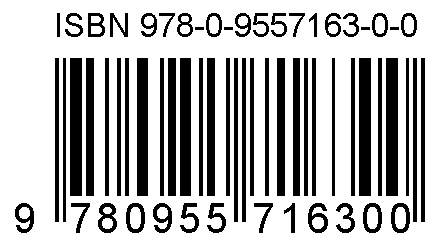Saturday, September 19, 2015

ISBN

Most books are issued a unique International Standard Book Number (ISBN) number. Often different formats of the same book will have different ISBN numbers. On a print book, you can usually find the ISBN on a barcode on the back cover.Most countries seem to have a national ISBN registration agency. In some countries this is a free service provided by a government agency. In other countries, this is operated by a commercial entity. In the United States, one company (R.R. Bowker LLC) has an apparent monopoly on issuing ISBN numbers which can cost \$125 for one (less if you buy in bulk).

The ISBN is 13 digits long if assigned starting in 2007, and 10 digits long if assigned before 2007. Each ISBN contains a check digit which is used for basic error detection We are going to build a few words in Factor to calculate the check digits and validate ISBNs.

We need to turn an ISBN (which might include spaces or dashes) into numeric digits:

```: digits ( str -- digits )
[ digit? ] filter string>digits ;```

For ISBN-10, the check digit is the sum of each of 10 digits multiplied by a weight (descending from 10 to 1) modulo 11.

```: isbn-10-check ( digits -- n )
0 [ 10 swap - * + ] reduce-index 11 mod ;```

For ISBN-13, the check digit is the sum of each of 13 digits multiplied by a weight (alternating between 1 and 3) modulo 10.

```: isbn-13-check ( digits -- n )
0 [ even? 1 3 ? * + ] reduce-index 10 mod ;```

We can validate an ISBN by grabbing the digits and running either the ISBN-10 or ISBN-13 check and verifying that the result is zero.

```: valid-isbn? ( str -- ? )
digits dup length {
{ 10 [ isbn-10-check ] }
{ 13 [ isbn-13-check ] }
} case 0 = ;```

The code (and some tests) for this is on my GitHub.